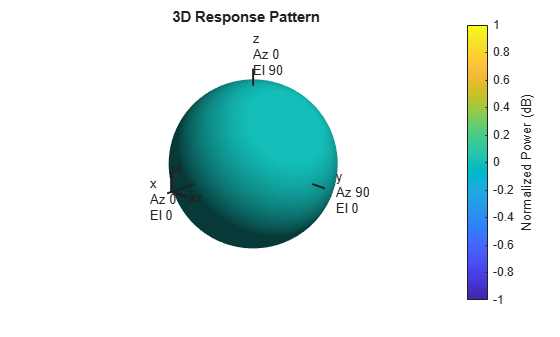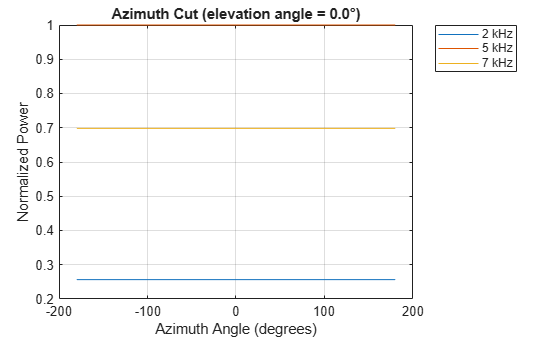Documentation

# step

System object: phased.IsotropicHydrophone
Package: phased

Voltage sensitivity of isotropic hydrophone

## Syntax

```resp = step(hydrophone,freq,ang) ```

## Description

### Note

Instead of using the `step` method to perform the operation defined by the System object™, you can call the object with arguments, as if it were a function. For example, ```y = step(obj,x)``` and `y = obj(x)` perform equivalent operations.

`resp = step(hydrophone,freq,ang)` returns the voltage sensitivity for the hydrophone at the specified operating frequencies and in the specified directions of arriving signals.

### Note

The object performs an initialization the first time the object is executed. This initialization locks nontunable properties (MATLAB) and input specifications, such as dimensions, complexity, and data type of the input data. If you change a nontunable property or an input specification, the System object issues an error. To change nontunable properties or inputs, you must first call the `release` method to unlock the object.

## Input Arguments

expand all

Isotropic hydrophone, specified as a `phased.IsotropicHydrophone` System object.

Example: `phased.IsotropicHydrophone`

Voltage sensitivity frequencies of hydrophone, specified as a positive real scalar or a real-valued 1-by-L vector of positive values. Units are in Hz.

Data Types: `double`

Direction of arriving signals, specified as a real-valued 1-by-M row vector or 2-by-M matrix. When `ang` is a 2-by-M matrix, each column of the matrix specifies the direction in the form `[azimuth;elevation]`. The azimuth angle must lie between –180° and 180°, inclusive. The elevation angle must lie between –90° and 90°, inclusive.

When `ang` is a 1-by-M row vector, each element specifies the azimuth angle of the arriving signal. In this case, the corresponding elevation angle is assumed to be zero.

Data Types: `double`

## Output Arguments

expand all

Voltage sensitivity of hydrophone, returned as a real-valued M-by-L matrix. M represents the number of angles specified in `ang`, and L represents the number of frequencies specified in `freq`. Units are in V/Pa.

## Examples

expand all

Examine the response and patterns of an isotropic hydrophone operating between 1 kHz and 10 kHz.

Set up the hydrophone parameters. Obtain the voltage sensitivity at five different elevation angles: -30�, -15�, 0�, 15� and 30�. All elevation angles are at 0&deg;. The sensitivities are computed at the signal frequency of 2 kHz.

```hydrophone = phased.IsotropicHydrophone('FrequencyRange',[1 10]*1e3); fc = 2e3; resp = hydrophone(fc,[0 0 0 0 0;-30 -15 0 15 30]);```

Draw a 3-D plot of the voltage sensitivity.

```pattern(hydrophone,fc,[-180:180],[-90:90],'CoordinateSystem','polar', ... 'Type','powerdb')```Examine the response and patterns of an isotropic hydrophone at three different frequencies. The hydrophone operates between 1 kHz and 10 kHz. Specify the voltage sensitivity as a vector.

Set up the hydrophone parameters and obtain the voltage sensitivity at 45° azimuth and 30° elevation. Compute the sensitivities at the signal frequencies of 2, 5, and 7 kHz.

```hydrophone = phased.IsotropicHydrophone('FrequencyRange',[1 10]*1e3, ... 'VoltageSensitivity',[-100 -90 -100]); fc = [2e3 5e3 7e3]; resp = hydrophone(fc,[45;30])```
```resp = 1×3 14.8051 29.2202 24.4152 ```

Draw a 2-D plot of the voltage sensitivity as a function of azimuth.

```pattern(hydrophone,fc,[-180:180],0,'CoordinateSystem','rectangular',... 'Type','power')```## Algorithms

The total sensitivity of a hydrophone is a combination of its frequency sensitivity and spatial sensitivity. `phased.IsotropicHydrophone` calculates both sensitivities using nearest neighbor interpolation, and then multiplies the sensitivities to form the total sensitivity.